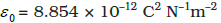# Coulombs Law

##### 693

Columbs law states that whenever two charges are placed in vicinity of each other, they create a force betwwen them

ie if two charges q1 and q2 are placed at a separation of distance r between them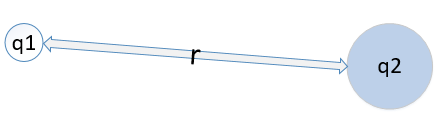Then Force between them is equal to :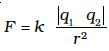Where k is constant k is equal to :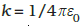where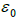is called permittivity of free space and its value is equal to :